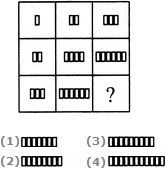# Non Verbal Reasoning - Figure Matrix - Discussion

### Discussion :: Figure Matrix - Section 2 (Q.No.2)

In each of the following questions, find out which of the answer figures (1), (2), (3) and (4) completes the figure matrix ?

2.

Select a suitable figure from the four alternatives that would complete the figure matrix.[A]. 1 [B]. 2 [C]. 3 [D]. 4

Explanation:

In each row (as well as in each column), the number of rectangles in the second figure is two times the number of rectangles in the first figure and the number of rectangles in the third figure is three times the number of rectangles in the first figure.

 Johnny said: (Apr 9, 2013) In 2nd row AND column, the rectangles are +2 the previous figure, not x2.

 Master Joe said: (Jun 23, 2016) The 2nd column and second row has a corresponding pattern which is +2 isn't it?